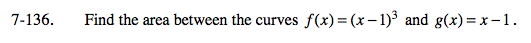### Home > CALC > Chapter 7 > Lesson 7.3.3 > Problem7-136

7-136.

Find the area between the curves f(x) = (x − 1)3 and g(x) = x − 1. Homework Help ✎How many points of intersection are there between these two graphs? How many integrals are required?

Within the bounds of integration, which function is on top and which is on the bottom?